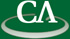## Invariant Lie polynomials in the representation of sl(2) and sl(3)

### Hu Jiaxiong, Queens University

January 7th, 2010 at 12:30pm in K9509.

```In this talk, I will focus on the nonassociative algebra of invariant
polynomials, which is rarely studied in invariant theory. I will introduce how
to compute the nonassociative invariants (invariant Lie polynomials) in the
representations of sl(2) and sl(3), where L is a free Lie algebra generated by
2 and 3 elements respectively. The rapid growth of the number of Lie invariants
of degree mr as a function of m, where r is the number of the free generators
(variables), suggests that the ring of the Lie invariants in r variables
probably can not be generated by a finite number of invariants (nobody proved it).
Due to heavy calculation task, computer algebra needs to come into play.

This is a joint work with Murray Bremner (University of Saskatchewan).
```